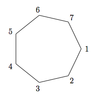# Question on subgroup and order of the elements

• MHB
• himynameJEF

#### himynameJEF

Let G be the group of symmetries (including flips) of the regular heptagon (7-gon).

View attachment 8446

As usual, we regard the elements of G as permutations of the set of vertex labels; thus, G ≤ S7.

(a) Let σ denote the rotation of the 7-gon that takes the vertex 1 to the vertex 2. Write down the cyclic subgroup R := ⟨σ⟩ as a set of elements of S7 in cycle notation.

(b) What are the orders of each of the elements of R?

does this mean R := ⟨( 1 2 3 4 5 6 7 )⟩?
and I am unsure how to answer part b)

thanks

#### Attachments

•Screen Shot 2018-10-01 at 3.37.31 am.png
1.8 KB · Views: 37

Let G be the group of symmetries (including flips) of the regular heptagon (7-gon).

As usual, we regard the elements of G as permutations of the set of vertex labels; thus, G ≤ S7.

(a) Let σ denote the rotation of the 7-gon that takes the vertex 1 to the vertex 2. Write down the cyclic subgroup R := ⟨σ⟩ as a set of elements of S7 in cycle notation.

(b) What are the orders of each of the elements of R?

does this mean R := ⟨( 1 2 3 4 5 6 7 )⟩?
and I am unsure how to answer part b)

thanks

Hi himynameJEF, welcome to MHB! ;)

Yes, that is correct for what $R$ stands for.
Which elements exactly are in $R$ though?
That is, what is for instance ( 1 2 3 4 5 6 7 )2?

As for (b), let's start with the order of ( 1 2 3 4 5 6 7 ).
What is it?

Hi himynameJEF, welcome to MHB! ;)

Yes, that is correct for what $R$ stands for.
Which elements exactly are in $R$ though?
That is, what is for instance ( 1 2 3 4 5 6 7 )2?

As for (b), let's start with the order of ( 1 2 3 4 5 6 7 ).
What is it?

hi! :)

( 1 2 3 4 5 6 7 )2 would be ( 1 3 5 7 2 4 6 )?

and that would be an order of 7?

thanks!

hi! :)

( 1 2 3 4 5 6 7 )2 would be ( 1 3 5 7 2 4 6 )?

and that would be an order of 7?

thanks!

Yep. (Nod)

So now we have 2 elements in $R$ that both have order 7.
How about ( 1 2 3 4 5 6 7 )3? (Wondering)

Yep. (Nod)

So now we have 2 elements in $R$ that both have order 7.
How about ( 1 2 3 4 5 6 7 )3? (Wondering)

thanks i understand it now! :)

also another question

Let H be any subgroup of G other than G itself. explain why H is cyclic?

since G is prime then |H| is 1 or 7. then H must equal G and it would be cyclic but the question says any other subgroup other than G so H must be {e}? is this cyclic? I am confused

thanks i understand it now! :)

also another question

Let H be any subgroup of G other than G itself. explain why H is cyclic?

since G is prime then |H| is 1 or 7. then H must equal G and it would be cyclic but the question says any other subgroup other than G so H must be {e}? is this cyclic? I am confused

Isn't the order of G 14?
That is, aren't there 14 symmetries with rotations and flips?

Isn't the order of G 14?
That is, aren't there 14 symmetries with rotations and flips?

so how would i explain this? and then determine the number of subgroups?

thanks!